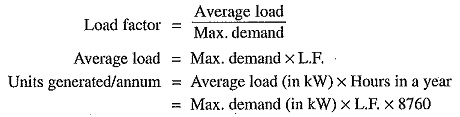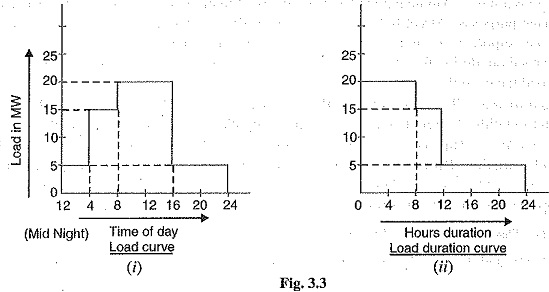The variable load problem has introduced the following terms and factors in power plant engineering:

1.Connected load : It is the sum of continuous ratings of all the equipment’s connected to supply system.

A power station supplies load to thousands of consumers. Each consumer has certain equipment installed in his premises. The sum of the continuous ratings of all the equipment’s in the consumer’s premises is the “connected load” of the consumer. For instance, if a consumer has connections of five 100-watt lamps and a power point of 500 watts, then connected load of the consumer is 5 x 100 + 500 = 1000 watts. The sum of the connected loads of all the consumers is the connected load to the power station.

2.Maximum demand : It is the greatest demand of load on the power station during a given period.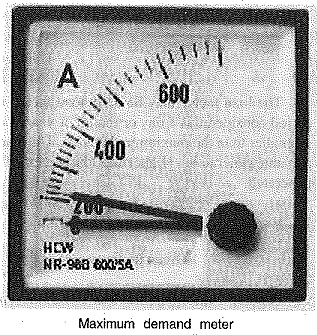The Variable Load Problem on the power station varies from time to time. The maximum of all the demands that have occurred during a given period (say a day) is the maximum demand. Thus referring back to the load curve of Fig. 3.2, the maximum demand on the power station during the day is 6 MW and it occurs at 6 P.M. Maximum demand is generally less than the connected load because all the consumers do not switch on their connected load to the system at a time. The knowledge of maximum demand is very important as it helps in determining the installed capacity of the station. The station must be capable of meeting the maximum demand.

3.Demand factor : It is the ratio of maximum demand on the power station to its connected load i.e.,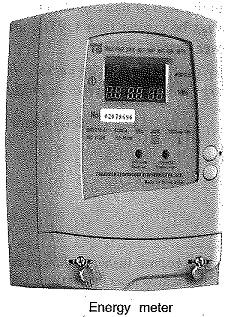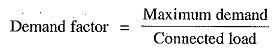The value of demand factor is usually less than 1. It is expected because maximum demand on the power station is generally less than the connected load. If the maximum demand on the power station is 80 MW and the connected load is 100 MW, then demand factor = 80/100 = 0.8. The knowledge of demand factor is vital in determining the capacity of the plant equipment.

4.Average Load :  The average of loads occurring on the power station in a given period (day or month or year) is known as average load or average demand.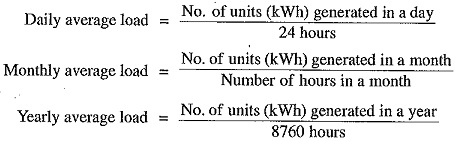5.Load factor : The ratio of average load to the maximum demand during a given period is known as load factor e.,If the plant is in operation for T hours,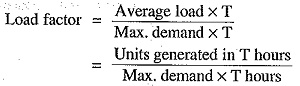The load factor may be daily load factor, monthly load factor or annual load factor if the time period considered is a day or month or year. Load factor is always less than 1 because average load is smaller than the maximum demand. The load factor plays key role in determining the overall cost per unit generated. Higher the load factor of the power station, lesser will be the cost per unit generated.

6.Diversity factor : The ratio of the sum of individual maximum demands to the maximum demand on power station is known as diversity factor e.,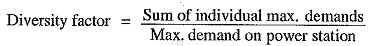A power station supplies Variable Load Problem to various types of consumers whose maximum demands generally do not occur at the same time. Therefore, the maximum demand on the power station is always less than the sum of individual maximum demands of the consumers. Obviously, diversity’ factor will always be greater than 1. The greater the diversity factor, the lesser is the cost of generation of power.

7.Plant capacity factor : It is the ratio of actual energy produced to the Maximum possible energy that could have been produced during a given period i.e.,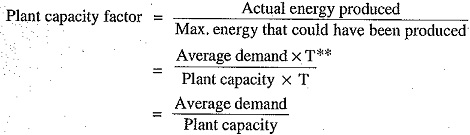Thus if the considered period is one year,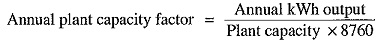The plant capacity factor is an indication of the reserve capacity of the plant. A power station is so designed that it has some reserve capacity for meeting the increased Variable Load Problem demand in future. Therefore, the installed capacity of the plant is always somewhat greater than the maximum demand on the plant.

Reserve capacity = Plant capacity — Max. demand

It is interesting to note that difference between load factor and plant capacity factor is an indication of reserve capacity. If the maximum demand on the plant is equal to the plant capacity, then load factor and plant capacity factor will have the same value. In such a case, the plant will have no reserve capacity.

8.Plant use factor : It is ratio of kWh generated to the product of plant capacity and the number of hours for which the plant was in operation i.e.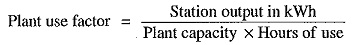Suppose a plant having installed capacity of 20 MW produces annual output of 7.35 x 106 kWh and remains in operation for 2190 hours in a year. Then,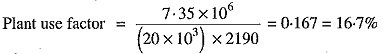### Units Generated per Annum

It is often required to find the kWh generated per annum from maximum demand and load factor. The procedure is as follows :